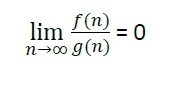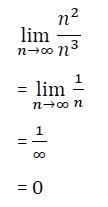# Little Oh Notation (o)

## Little o Notations

There are some other notations present except the Big-Oh, Big-Omega and Big-Theta notations. The little o notation is one of them.

Little o notation is used to describe an upper bound that cannot be tight. In other words, loose upper bound of f(n).

Let f(n) and g(n) are the functions that map positive real numbers. We can say that the function f(n) is o(g(n)) if for any real positive constant c, there exists an integer constant n0 ≤ 1 such that f(n) > 0.

## Mathematical Relation of Little o notation

Using mathematical relation, we can say that f(n) = o(g(n)) means,## Example on little o asymptotic notation

If f(n) = n2 and g(n) = n3 then check whether f(n) = o(g(n)) or not.The result is 0, and it satisfies the equation mentioned above. So we can say that f(n) = o(g(n)).